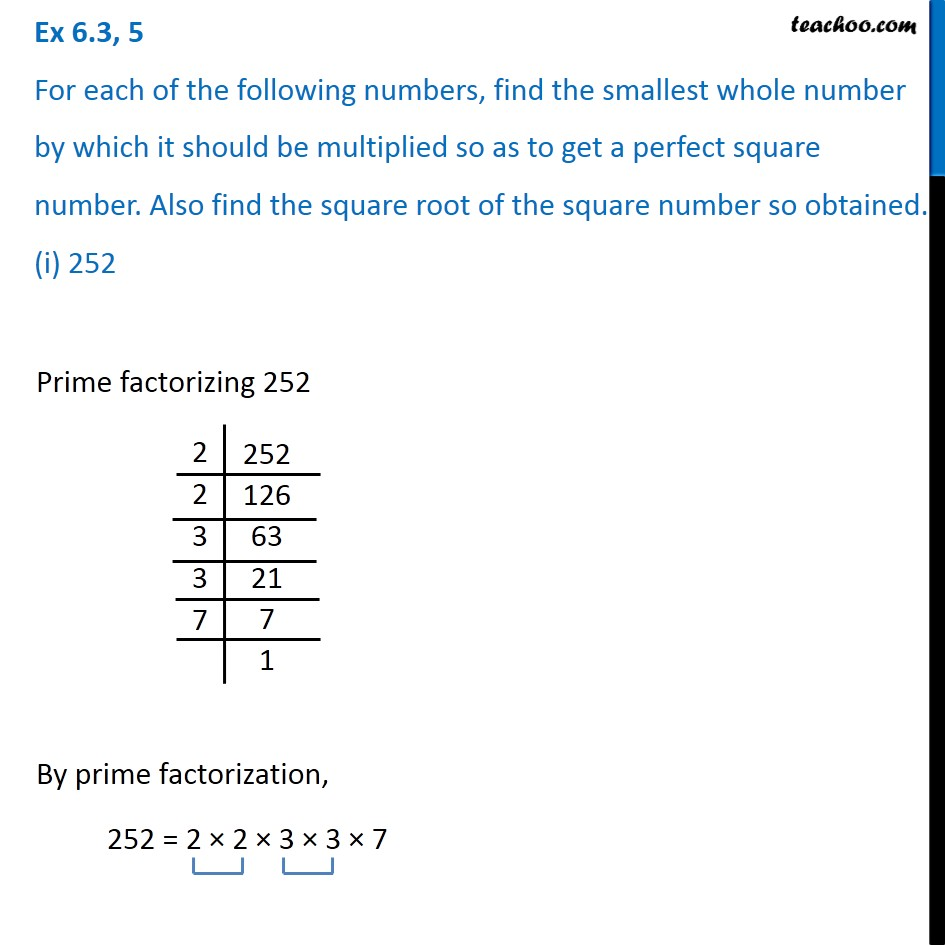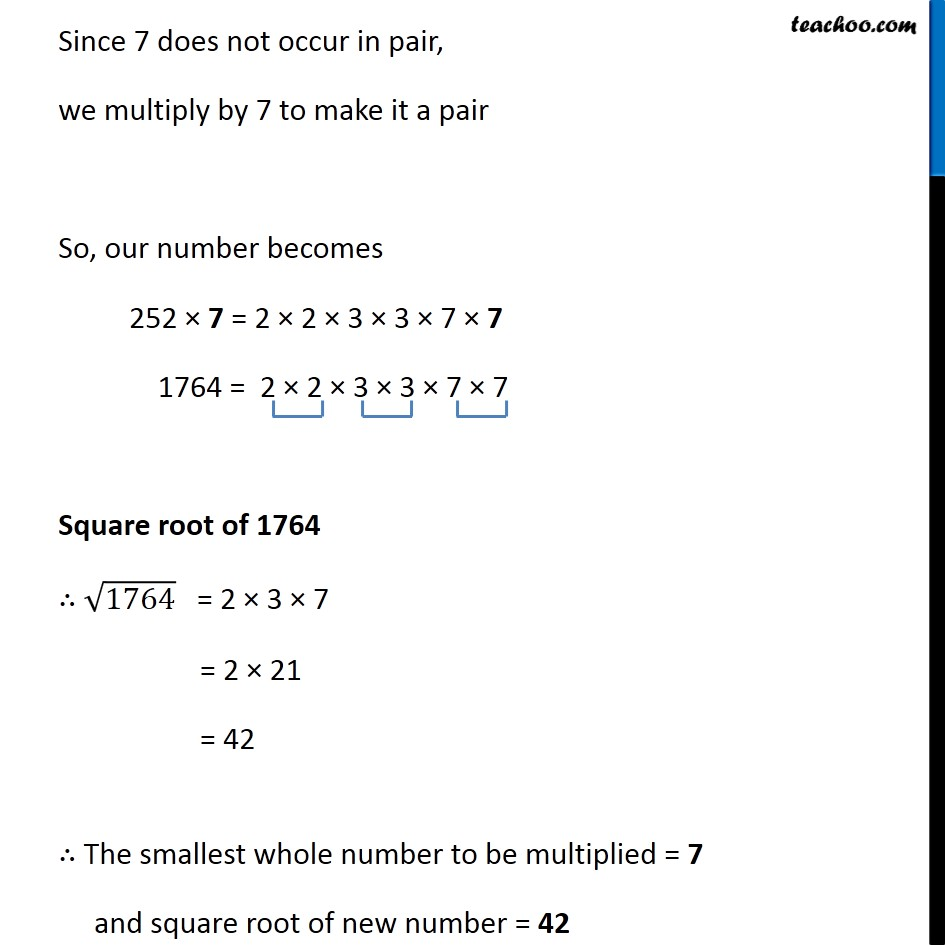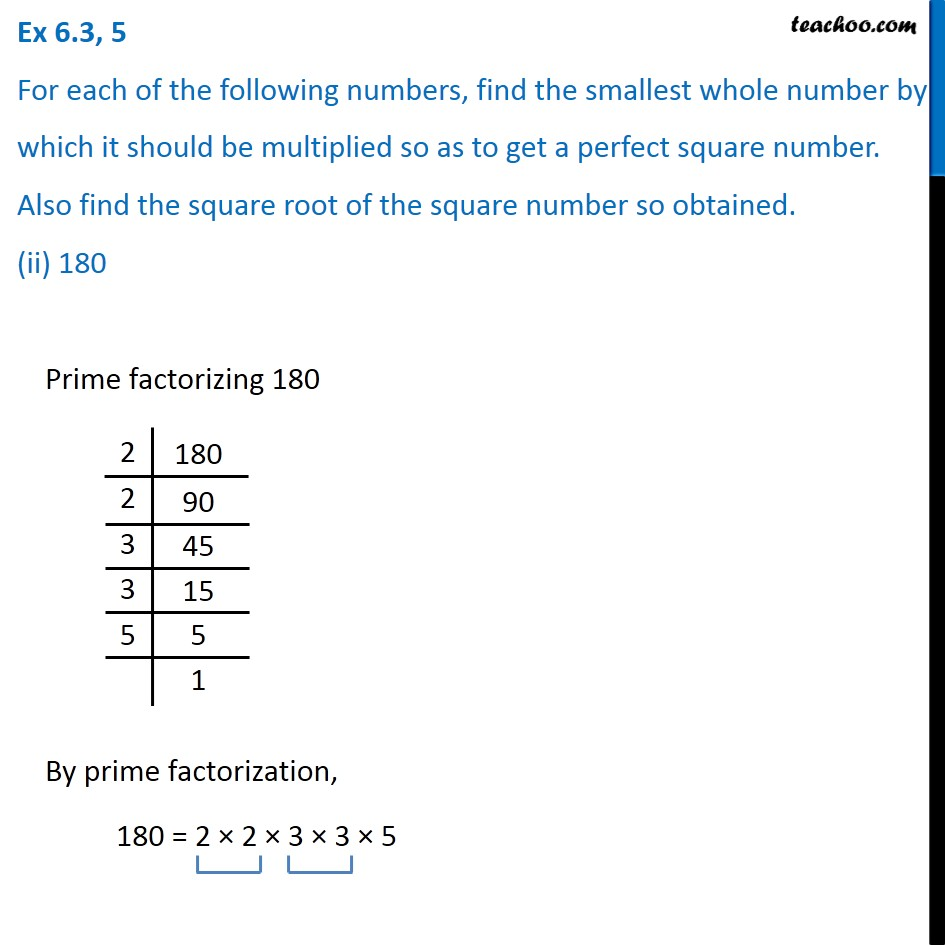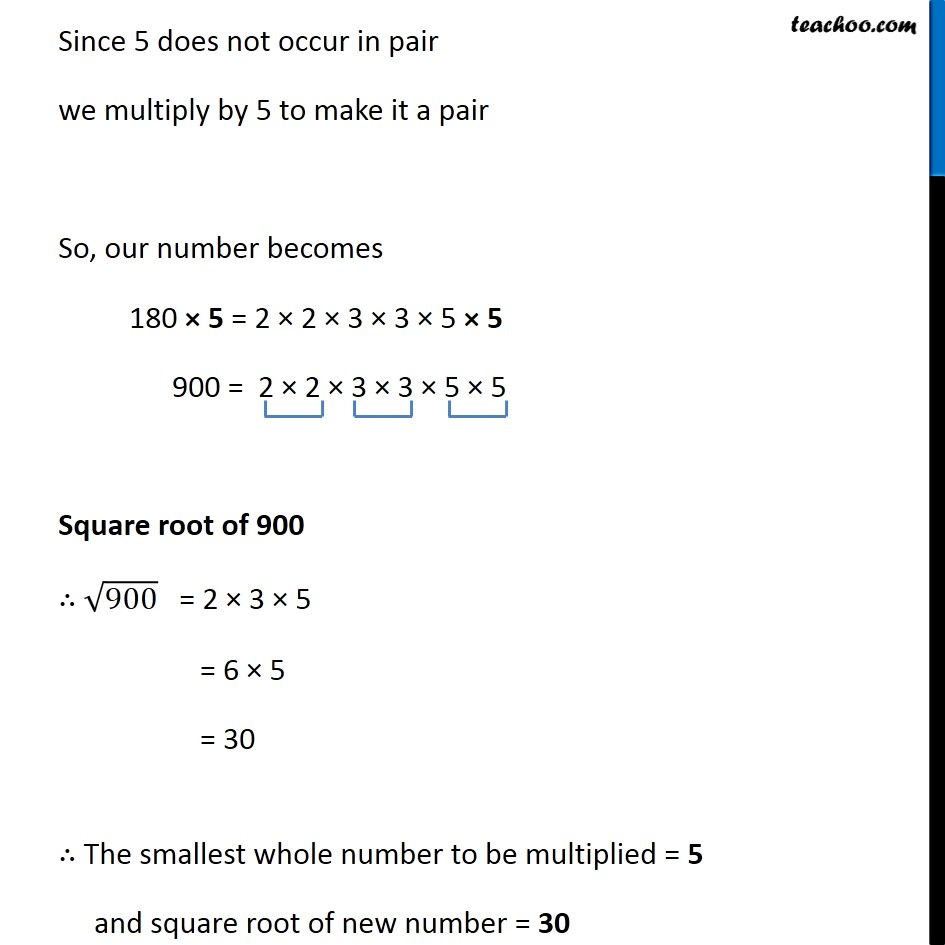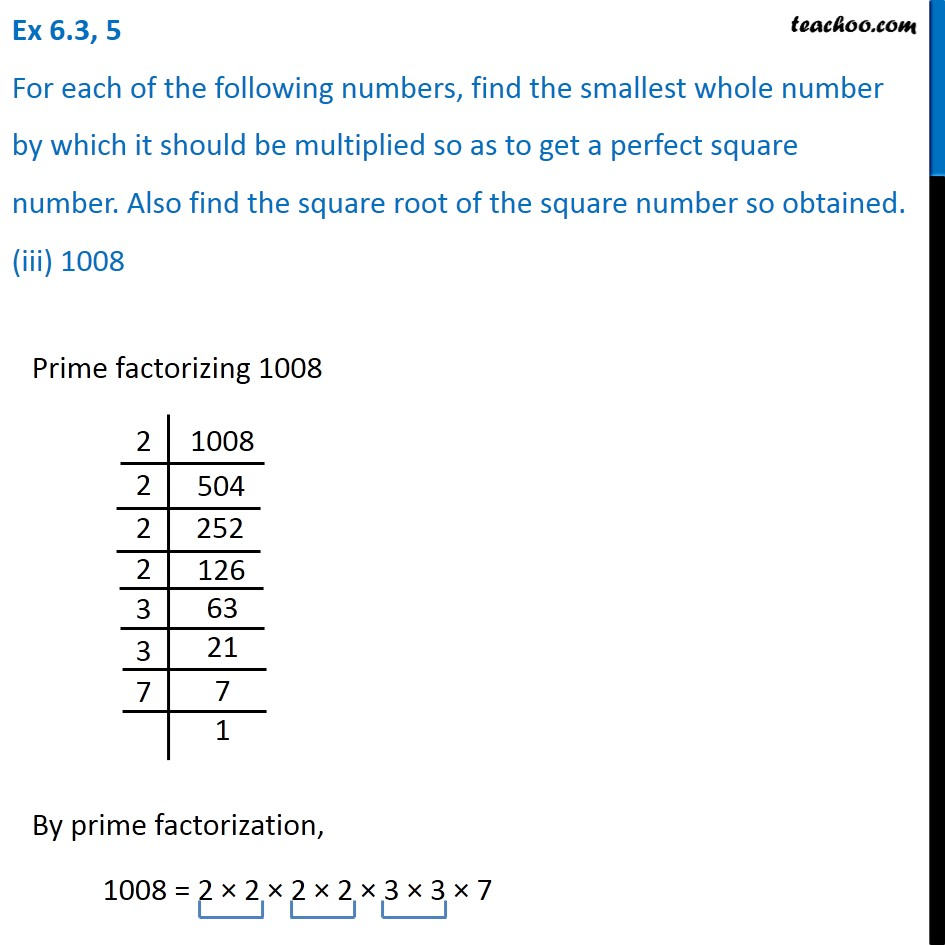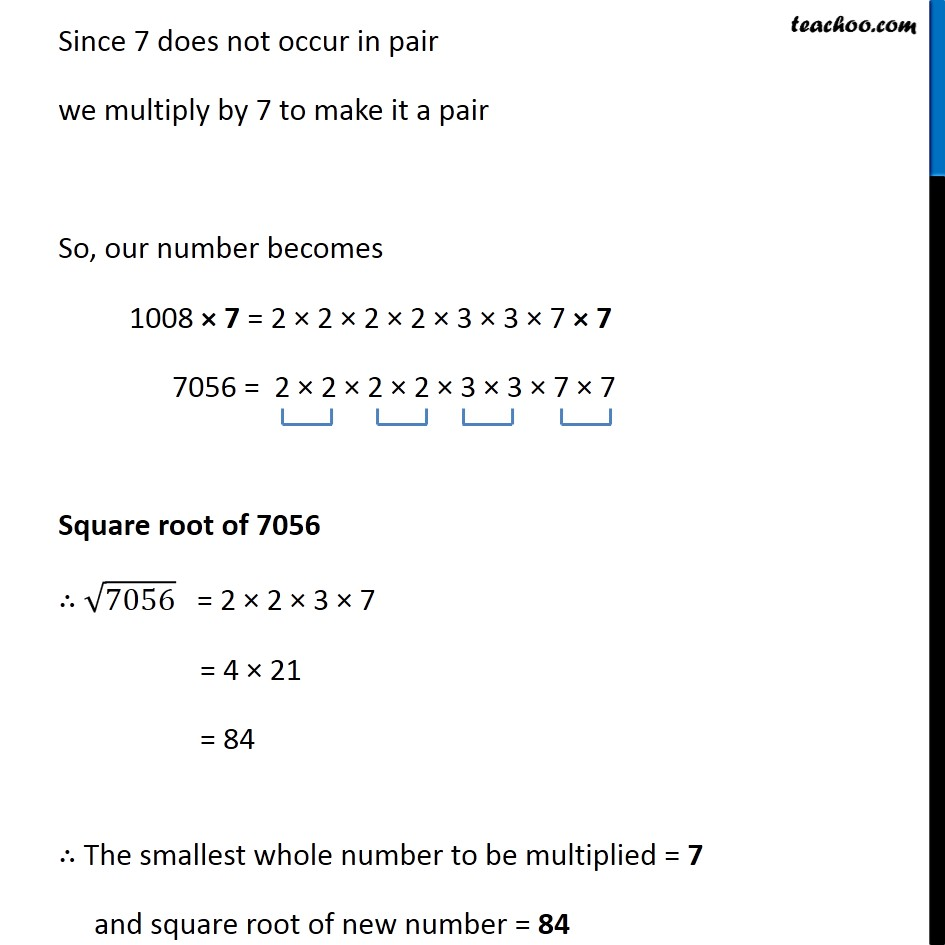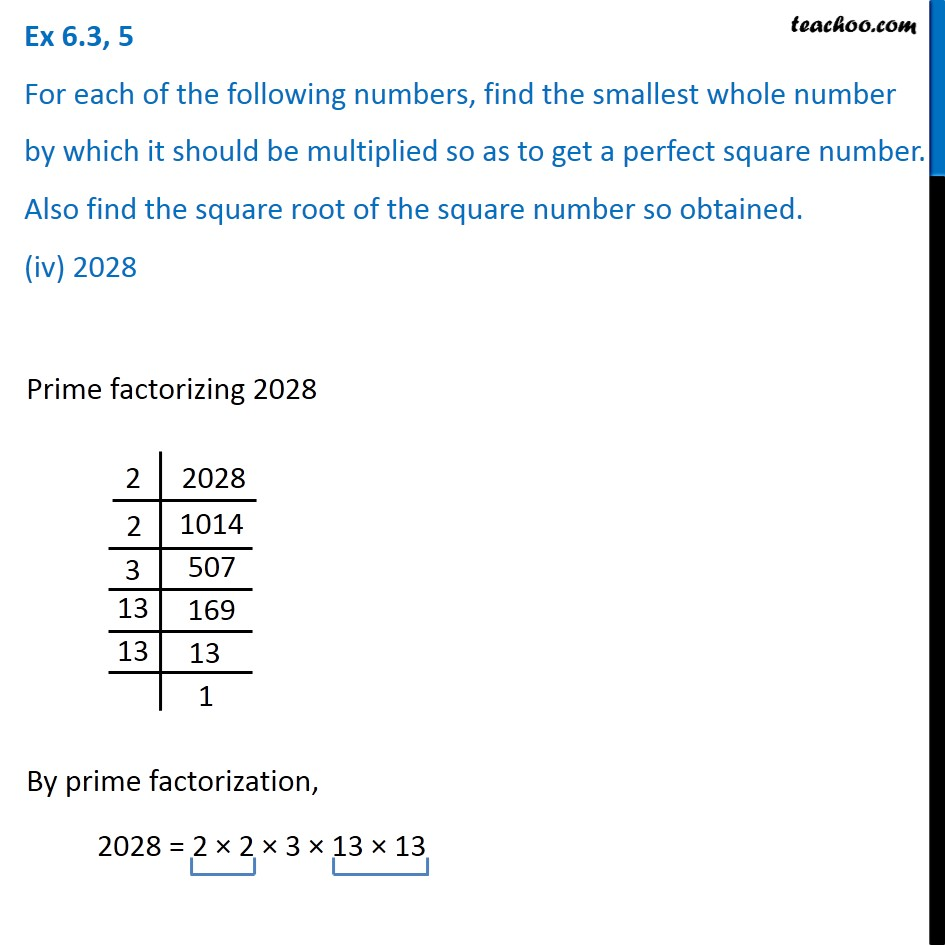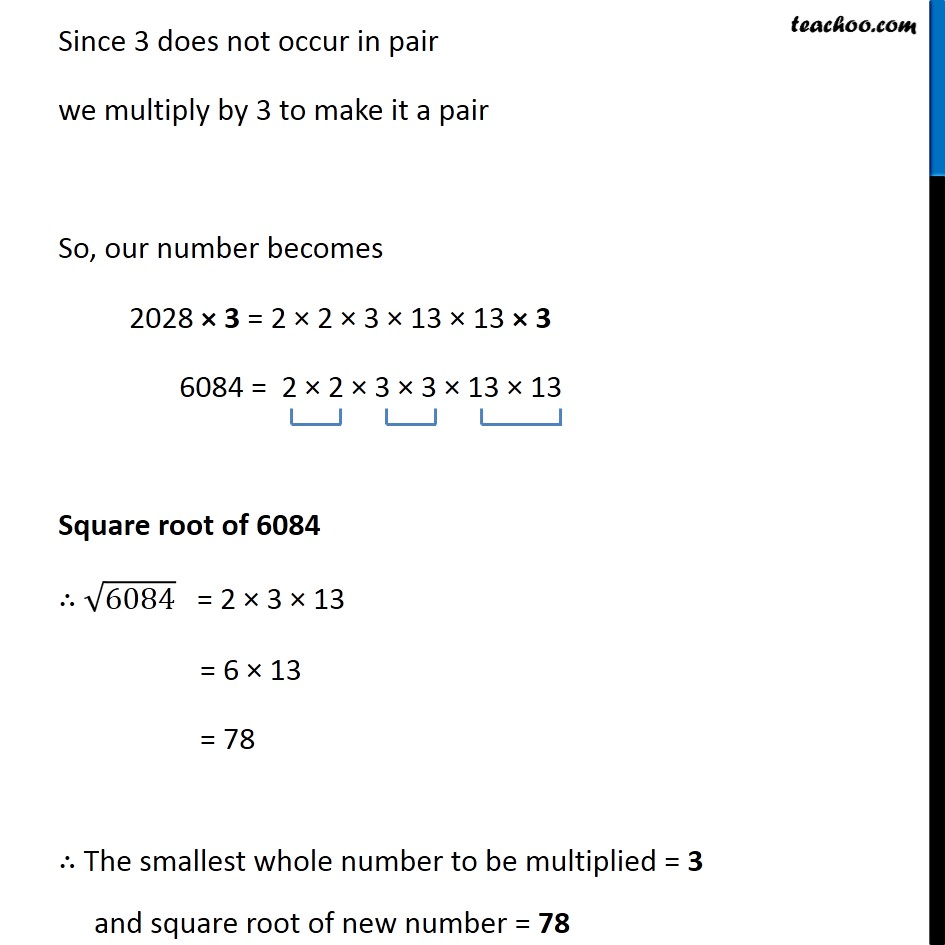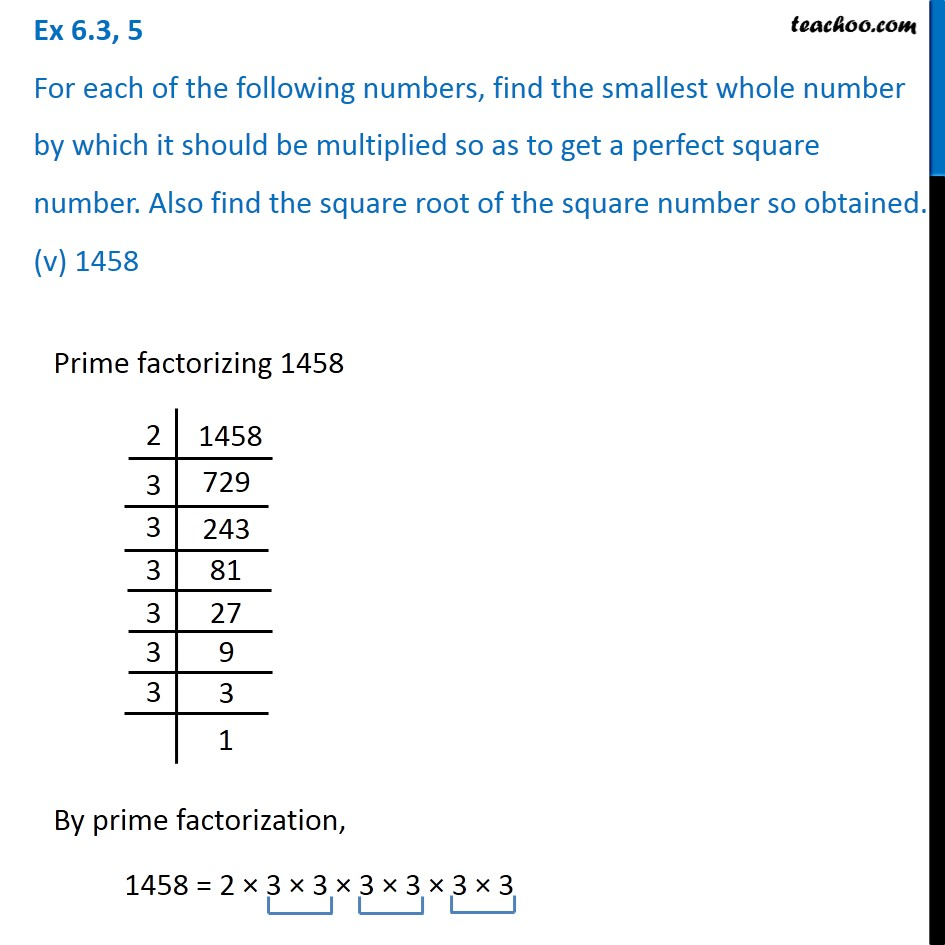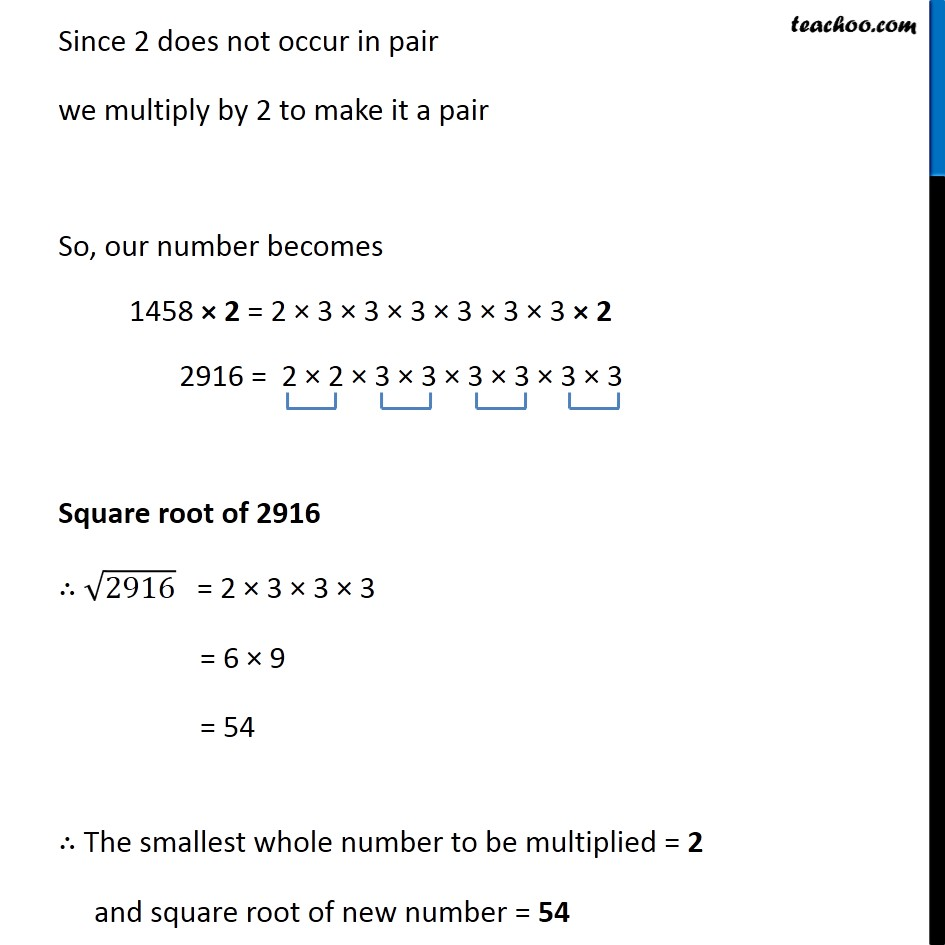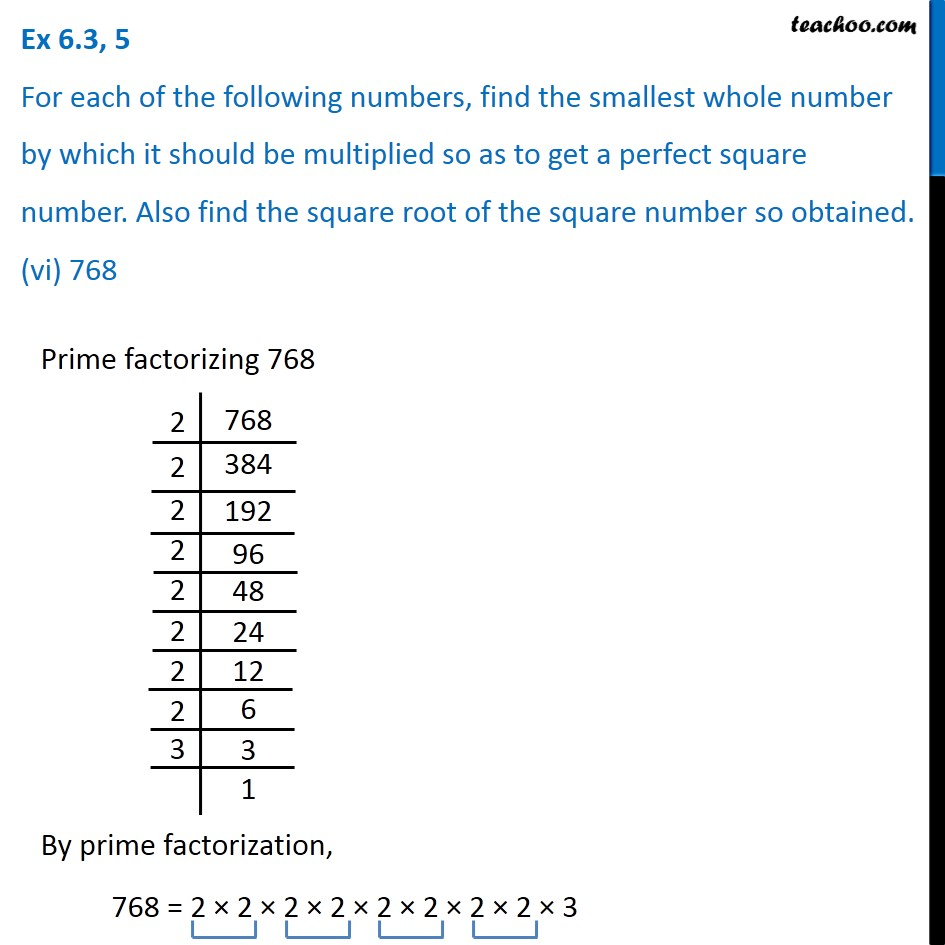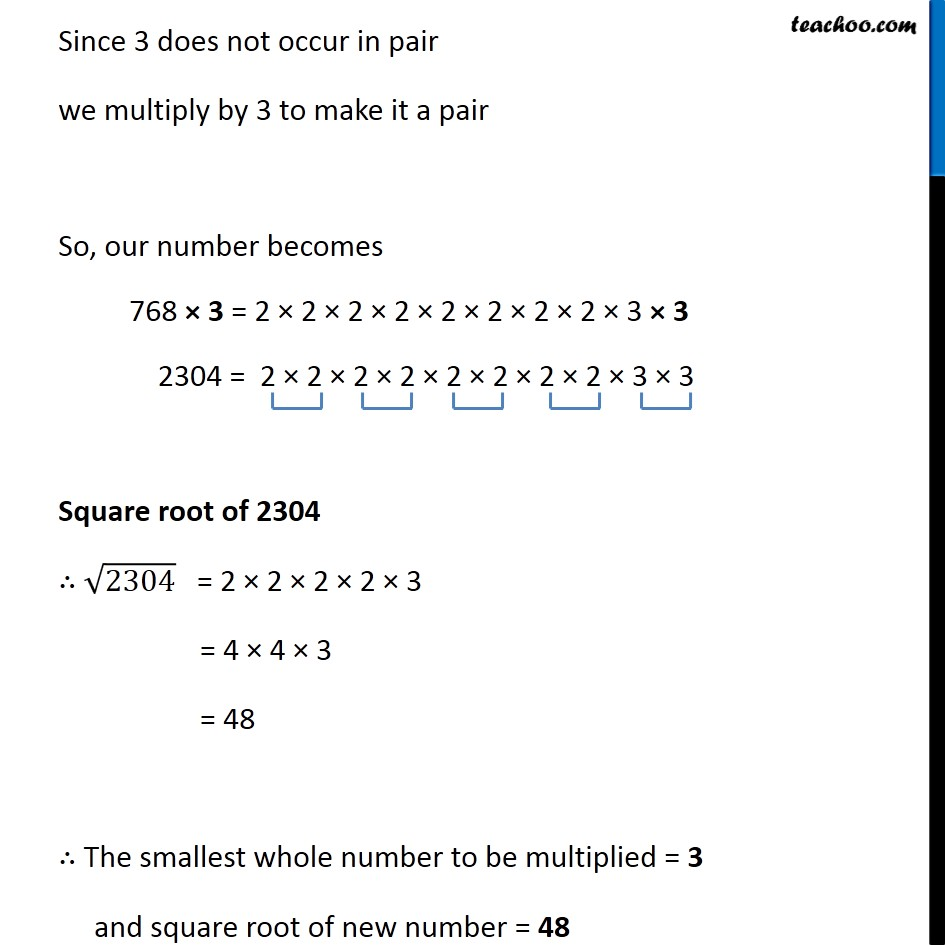Subscribe to our Youtube Channel - https://you.tube/teachoo

1. Chapter 6 Class 8 Squares and Square Roots
2. Serial order wise
3. Ex 6.3

Transcript

Ex 6.3, 5 For each of the following numbers, find the smallest whole number by which it should be multiplied so as to get a perfect square number. Also find the square root of the square number so obtained. (i) 252 Prime factorizing 252 By prime factorization, 252 = 2 × 2 × 3 × 3 × 7 Since 7 does not occur in pair, we multiply by 7 to make it a pair So, our number becomes 252 × 7 = 2 × 2 × 3 × 3 × 7 × 7 1764 = 2 × 2 × 3 × 3 × 7 × 7 Square root of 1764 ∴ √1764 = 2 × 3 × 7 = 2 × 21 = 42 ∴ The smallest whole number to be multiplied = 7 and square root of new number = 42 Ex 6.3, 5 For each of the following numbers, find the smallest whole number by which it should be multiplied so as to get a perfect square number. Also find the square root of the square number so obtained. (ii) 180 Prime factorizing 180 By prime factorization, 180 = 2 × 2 × 3 × 3 × 5 Since 5 does not occur in pair we multiply by 5 to make it a pair So, our number becomes 180 × 5 = 2 × 2 × 3 × 3 × 5 × 5 900 = 2 × 2 × 3 × 3 × 5 × 5 Square root of 900 ∴ √900 = 2 × 3 × 5 = 6 × 5 = 30 ∴ The smallest whole number to be multiplied = 5 and square root of new number = 30 Ex 6.3, 5 For each of the following numbers, find the smallest whole number by which it should be multiplied so as to get a perfect square number. Also find the square root of the square number so obtained. (iii) 1008 Prime factorizing 1008 By prime factorization, 1008 = 2 × 2 × 2 × 2 × 3 × 3 × 7 Since 7 does not occur in pair we multiply by 7 to make it a pair So, our number becomes 1008 × 7 = 2 × 2 × 2 × 2 × 3 × 3 × 7 × 7 7056 = 2 × 2 × 2 × 2 × 3 × 3 × 7 × 7 Square root of 7056 ∴ √7056 = 2 × 2 × 3 × 7 = 4 × 21 = 84 ∴ The smallest whole number to be multiplied = 7 and square root of new number = 84 Ex 6.3, 5 For each of the following numbers, find the smallest whole number by which it should be multiplied so as to get a perfect square number. Also find the square root of the square number so obtained. (iv) 2028 Prime factorizing 2028 By prime factorization, 2028 = 2 × 2 × 3 × 13 × 13 Since 3 does not occur in pair we multiply by 3 to make it a pair So, our number becomes 2028 × 3 = 2 × 2 × 3 × 13 × 13 × 3 6084 = 2 × 2 × 3 × 3 × 13 × 13 Square root of 6084 ∴ √6084 = 2 × 3 × 13 = 6 × 13 = 78 ∴ The smallest whole number to be multiplied = 3 and square root of new number = 78 Ex 6.3, 5 For each of the following numbers, find the smallest whole number by which it should be multiplied so as to get a perfect square number. Also find the square root of the square number so obtained. (v) 1458 Prime factorizing 1458 By prime factorization, 1458 = 2 × 3 × 3 × 3 × 3 × 3 × 3 Since 2 does not occur in pair we multiply by 2 to make it a pair So, our number becomes 1458 × 2 = 2 × 3 × 3 × 3 × 3 × 3 × 3 × 2 2916 = 2 × 2 × 3 × 3 × 3 × 3 × 3 × 3 Square root of 2916 ∴ √2916 = 2 × 3 × 3 × 3 = 6 × 9 = 54 ∴ The smallest whole number to be multiplied = 2 and square root of new number = 54 Ex 6.3, 5 For each of the following numbers, find the smallest whole number by which it should be multiplied so as to get a perfect square number. Also find the square root of the square number so obtained. (vi) 768 Prime factorizing 768 By prime factorization, 768 = 2 × 2 × 2 × 2 × 2 × 2 × 2 × 2 × 3 Since 3 does not occur in pair we multiply by 3 to make it a pair So, our number becomes 768 × 3 = 2 × 2 × 2 × 2 × 2 × 2 × 2 × 2 × 3 × 3 2304 = 2 × 2 × 2 × 2 × 2 × 2 × 2 × 2 × 3 × 3 Square root of 2304 ∴ √2304 = 2 × 2 × 2 × 2 × 3 = 4 × 4 × 3 = 48 ∴ The smallest whole number to be multiplied = 3 and square root of new number = 48

Ex 6.3